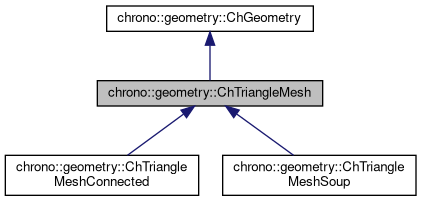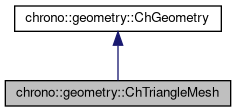chrono::geometry::ChTriangleMesh Class Referenceabstract

## Description

Base class for triangle meshes.

#include <ChTriangleMesh.h>

Inheritance diagram for chrono::geometry::ChTriangleMesh:[legend]
Collaboration diagram for chrono::geometry::ChTriangleMesh:[legend]

## Public Member Functions

virtual void addTriangle (const ChVector<> &vertex0, const ChVector<> &vertex1, const ChVector<> &vertex2)=0
Add a triangle to this triangle mesh, by specifying the three coordinates.

virtual void addTriangle (const ChTriangle &atriangle)=0
Add a triangle to this triangle mesh, by specifying a ChTriangle.

virtual int getNumTriangles () const =0

virtual ChTriangle getTriangle (int index) const =0
Get the n-th triangle in mesh.

virtual void Clear ()=0
Clear all data.

virtual void Transform (const ChVector<> displ, const ChMatrix33<> rotscale)=0
Transform all vertexes, by displacing and rotating (rotation via matrix, so also scaling if needed)

virtual void Transform (const ChVector<> displ, const ChQuaternion<> mquat=ChQuaternion<>(1, 0, 0, 0))
Transform all vertexes, by displacing and rotating (rotation via matrix, so also scaling if needed)

virtual GeometryType GetClassType () const override
Get the class type as unique numerical ID (faster than using ChronoRTTI mechanism). More...

virtual void GetBoundingBox (double &xmin, double &xmax, double &ymin, double &ymax, double &zmin, double &zmax, ChMatrix33<> *Rot=NULL) const override
Compute bounding box. More...

virtual void CovarianceMatrix (ChMatrix33<> &C) const override
Compute the 3x3 covariance matrix (only the diagonal and upper part) It should be overridden by inherited classes.

virtual int GetManifoldDimension () const override
This is a surface.

virtual void ArchiveOUT (ChArchiveOut &marchive) override
Method to allow serialization of transient data to archives.

virtual void ArchiveIN (ChArchiveIn &marchive) override
Method to allow de-serialization of transient data from archives.Public Member Functions inherited from chrono::geometry::ChGeometry
ChGeometry (const ChGeometry &source)

virtual ChGeometryClone () const =0
"Virtual" copy constructor.

virtual void InflateBoundingBox (double &xmin, double &xmax, double &ymin, double &ymax, double &zmin, double &zmax, ChMatrix33<> *Rot=NULL) const
Enlarge a previous existing bounding box. More...

virtual double Size () const
Returns the radius of the sphere which can enclose the geometry.

virtual ChVector Baricenter () const
Compute center of mass It should be overridden by inherited classes.

virtual void Update ()
Generic update of internal data. More...Public Types inherited from chrono::geometry::ChGeometry
enum  GeometryType {
NONE, SPHERE, BOX, CYLINDER,
TRIANGLE, CAPSULE, CONE, LINE,
LINE_ARC, LINE_BEZIER, LINE_CAM, LINE_PATH,
LINE_POLY, LINE_SEGMENT, ROUNDED_BOX, ROUNDED_CYLINDER,
ROUNDED_CONE, TRIANGLEMESH, TRIANGLEMESH_CONNECTED, TRIANGLEMESH_SOUP
}
Enumeration of geometric objects.

## ◆ GetBoundingBox()

 virtual void chrono::geometry::ChTriangleMesh::GetBoundingBox ( double & xmin, double & xmax, double & ymin, double & ymax, double & zmin, double & zmax, ChMatrix33<> * Rot = NULL ) const
inlineoverridevirtual

Compute bounding box.

If a matrix Rot is not null, it should compute bounding box along the rotated directions represented by that transformation matrix Rot. It must be overridden by inherited classes.

Reimplemented from chrono::geometry::ChGeometry.

## ◆ GetClassType()

 virtual GeometryType chrono::geometry::ChTriangleMesh::GetClassType ( ) const
inlineoverridevirtual

Get the class type as unique numerical ID (faster than using ChronoRTTI mechanism).

Each inherited class must return an unique ID.

Reimplemented from chrono::geometry::ChGeometry.

Reimplemented in chrono::geometry::ChTriangleMeshConnected, and chrono::geometry::ChTriangleMeshSoup.

The documentation for this class was generated from the following file:
• /builds/uwsbel/chrono/src/chrono/geometry/ChTriangleMesh.h